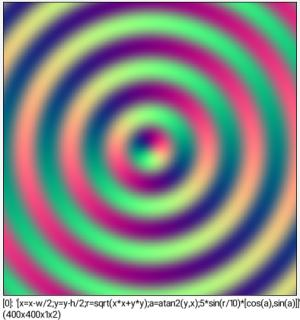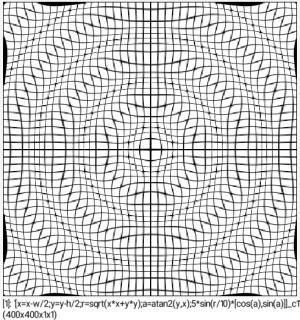G'MIC - GREYC's Magic for Image Computing: A Full-Featured Open-Source Framework for Image Processing# A Full-Featured Open-Source Framework for Image Processing

## Latest stable version: 3.1.5

Reference

 Table of Contents  ▸  List of Commands  ▸  Input / Output  ▸  display_warp ◀  display_tensors    |    echo  ▶

# display_warp

• _cell_size>0

## Description:

Render selected 2D warping fields.

(equivalent to shortcut command dw).

cell_size=15.

## Example of use:

\$ gmic 400,400,1,2,'x=x-w/2;y=y-h/2;r=sqrt(x*x+y*y);a=atan2(y,x);5*sin(r/10)*[cos(a),sin(a)]' +display_warp 10Command: 400,400,1,2,'x=x-w/2;y=y-h/2;r=sqrt(x*x+y*y);a=atan2(y,x);5*sin(r/10)*[cos(a),sin(a)]' +display_warp 10Command: 400,400,1,2,'x=x-w/2;y=y-h/2;r=sqrt(x*x+y*y);a=atan2(y,x);5*sin(r/10)*[cos(a),sin(a)]' +display_warp 10
G'MIC - GREYC's Magic for Image Computing: A Full-Featured Open-Source Framework for Image Processing